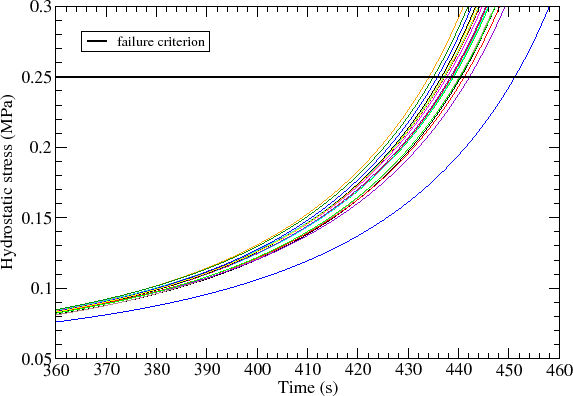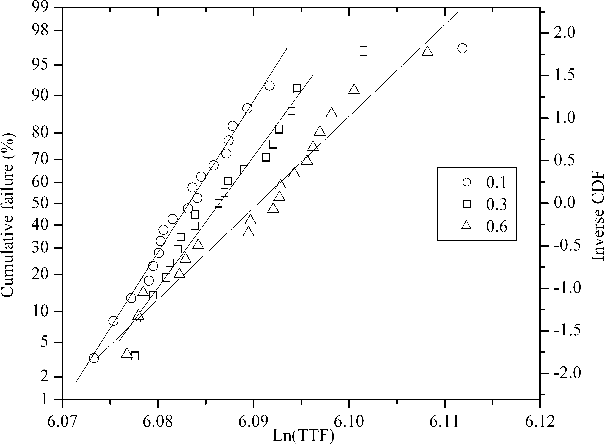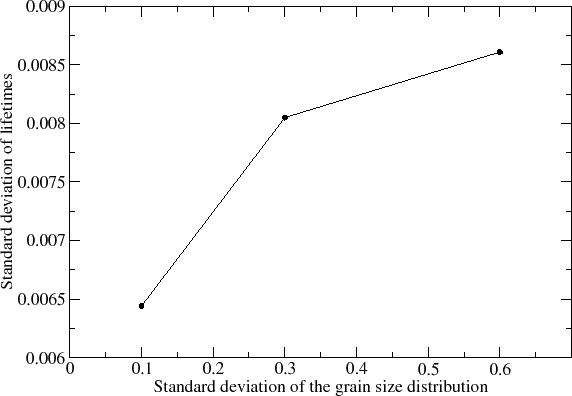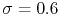Next: 6. Conclusion and Outlook Up: 5.6 Effect of Microstructure Previous: 5.6.3 Sites of Void

Figure 5.40 shows the hydrostatic tensile stress development for the structures with a grain size standard deviation of 0.3. The stress peak value follows the peak of the trapped vacancy concentration and is located at the intersection of grain boundaries with the capping layer, as shown in Figure 5.39.

Collecting the times to failure from Figure 5.40 and calculating the cumulative failure percentages results in the distributions of electromigration lifetimes shown in Figure 5.41. The lifetimes are fitted by lognormal distributions. The mean time to failure is determined by the 50% cumulative failure mark, and the slope of the curves corresponds to the inverse of the standard deviation of lifetimes. The obtained standard deviations are 0.0065, 0.0080, and 0.0085 for the grain size distributions with standard deviation of 0.1, 0.3, and 0.6, respectively.When the grain size distribution exhibits a smaller standard deviation the corresponding interconnect lines have a more uniform distribution of the grains. As a consequence, the stress build-up has smaller variations yielding a smaller standard deviation of the electromigration lifetimes. On the other hand, increasing the grain size standard deviation, the lines exhibit significant differences in the grain structures. This leads to larger variations of stress development. Thus, a bigger standard deviation of electromigration lifetimes is expected. The increase of the standard deviation of the electromigration lifetime distribution as a function of the standard deviation of the distribution of grain sizes is shown in Figure 5.42.The standard deviations of the electromigration lifetimes are very small compared to those frequently observed in experiments . Several factors can explain this behavior. First, for convenience, a small value of stress threshold as failure criterion is used to determine the interconnect lifetime. The complexity of the physical model associated with the numerical features posed by the microstructure makes the simulations inconveniently demanding. As can be seen from Figure 5.40, the variation of the lifetimes can be more pronounced for higher stress thresholds. Second, the simulation parameters and material properties are independent of the grain distribution. This means that mechanical properties and diffusivities, for example, are equal and constant for all simulated structures. This is clearly not the case in real experiments, as it is well known that material properties vary according to the grain network. It is expected that atomic diffusion along the copper/capping layer interface changes from grain to grain, inducing a flux divergence at the corresponding grain boundary. Moreover, the diffusivities are different from line to line as the grain distribution varies. Therefore, given the above simplifications, the small standard deviations obtained from the simulations should be expected.

Nevertheless, these results present important features which are worth a careful analysis. As a consequence of the larger lifetime standard deviation for the lines with, the early failures (failure percentages lower than 1% in Figure 5.41) occur at shorter times, even though the median lifetime is higher for these lines. This observation is of great importance to the extrapolation of accelerated test results to normal use conditions. This can be readily seen from an inspection of equation (1.23), which shows that the estimation of the real lifetimes has an exponential dependence on the standard deviation of lifetimes obtained by the accelerated tests.

Considering that the reliability assessment of a given technology is determined by the early failures, the understanding of the lifetime distribution becomes crucial. This means that the improvement of processes, and the choice of adequate materials, aiming the improvement of a given interconnect technology requires not only the increase of the median lifetime of an interconnect structure, but also the reduction of the corresponding standard deviation.Next: 6. Conclusion and Outlook Up: 5.6 Effect of Microstructure Previous: 5.6.3 Sites of Void

R. L. de Orio: Electromigration Modeling and Simulation